# Numerical Investigation of Heat Transfer and Pressure Force from Multiple Jets Impinging on a Moving Flat Surface

Numerical Investigation of Heat Transfer and Pressure Force from Multiple Jets Impinging on a Moving Flat Surface
Turbomachinery and Fluid Dynamics Laboratory, Ostwestfalen-Lippe University of Applied Science, Lemgo 32657, Germany

Institute for Multiphase Processes & Center for Biomedical Engineering, Leibniz University Hannover, Hannover 30167, Germany

Corresponding Author Email:
chitsazan@imp.uni-hannover.de
Page:
601-610
|
DOI:
https://doi.org/10.18280/ijht.380304
20 September 2019
|
Accepted:
12 July 2020
|
Published:
15 October 2020
| Citation

OPEN ACCESS

Abstract:

In this paper extensive numerical investigation of the heat transfer characteristics and the pressure force of jet impingement from the single row and multiple rows on a fixed and moving flat surface are reported. The computations were carried out over a wide range of parameters: relative nozzle-to-surface distance (H/d) from 0.5 to 6, relative nozzle to nozzle distances (S/d) from 4 to 10, jet angle from 45° to 90°, relative velocity ratio (Vplate/Vj) i.e. ratio of surface velocity to jet velocity from 0 to 1. The jet Reynolds number (Re) of 2,500, 3,400, 10,000, 20,000, and 23,000 and the number of jet rows of 1, 2, 4, and 8 have been used. It was found that the numerical accuracy by SST k-ω model is reasonably high to allow for a discussion of the main flow and heat transfer characteristics. The jet impingement heat transfer performance is generally enhanced with the increase of jet Reynolds number and jet angle and with the decrease of surface distance (H/d), jet distance (S/d) and the relative velocity ratio (Vplate/Vj) within the range examined. The pressure force coefficients on the impingement surface are relatively insensitive to Re number and the velocity ratio within the range examined, while it has highly dependent on H/d, S/d and jet angle. For multiple rows of aligned jet holes, the flow pattern exhibited a different shape due to the different intensity of the interference between adjacent air jets. The effect of multiple rows with regards to the impact on average Nu and pressure force coefficient for different geometry variations such as Re, H/d, S/d, VR and ɵ is negligible compared to the single row by approximately 9 and 13% in average respectively. Based on the computed results, equations of dimensionless parameters are correlated.

Keywords:

jet impingement, heat transfer, pressure force, multiple rows, jet angle, surface motion

1. Introduction

The phenomena for single jets are investigated extensively but are of limited use to industrial applications. Turbulent impinging jets are used in a variety of industrial applications involving cooling (e.g. hot rolled steel), heating and drying of flat sheets (e.g. tiles, tissues, paper, wood veneer). Depending on the actual process, the multiple arrays of jets with different geometries and angles impinge on the moving flat surface in order to provide the best configuration for convective heat and mass transfer as well as forces, due to the jet flow acting on the sheets.

The main flow patterns for multiple jets are shown in Figure 1. The fluid exits the nozzle with uniform velocity and a jet starts to evolve which is fully developed after approximately seven diameters. Before impinging on the surface, the jet flow is decelerated and the pressure rises in the stagnation zone until the impact on the surface (impingement zone). A wall jet evolves which is first accelerated and then decelerated (wall jet region). The wall jets collide causing a recirculation flow (fountain flow). There are shear layers in the free jet region as well as in the wall jet region (to the wall and to the adjacent fluid). In the impingement zone, there are normal stresses as well as shear stresses. The heat and mass transfer at the target plate is strongly influenced by these flow features. Geometrical parameters are the nozzle diameter d, the jet to jet distance S and the separation distance H.

## 1.jpg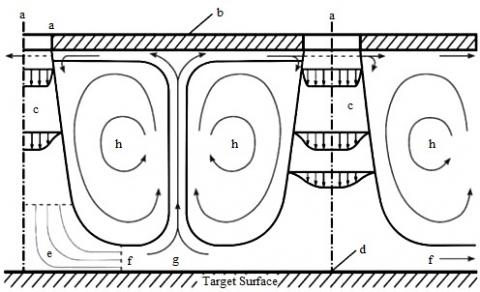Figure 1. Complex flow pattern within an array of impinging jets due to jet-to-jet interference: a – orifice, b –impingement plate, c – free jet, d – stagnation point, e – stagnation zone, f – decelerated flow, g – recirculating flow, h – vortices 

A comprehensive review of the heat transfer characteristics of systems of multiple impinging jets is presented by Weigand and Spring . An extensive survey of the heat transfer and flow phenomena associated with jet impingement is in Zuckerman and Lior . With decreased jet to jet distances or a small separation distance, the jet interaction increases significantly and the heat transfer rates can differ significantly from those of a single impinging jet.

Bu et al.  observed two peaks in the local Nusselt number distribution in the chordwise direction for two rows of jet holes due to the weak interference between adjacent air jets and only one peak for three rows of jet holes due to strong interference between adjacent air jets. Patil and Vedula  found that the peak Nusselt values with a single row and two rows of jet impinging on a concave surface are similar. The single row gives higher Nusselt values as compared to two rows with the same mass flow rate. Wae-haeey et al.  performed a heat transfer correlation for an impinging jet array discharged from pipe nozzles based on the peak of the average Nu number occurring at H/d = 4 in contrast to the case of impinging jet array discharged from orifice nozzles, in which the average Nu number decreased monotonically with jet to surface distance. Li et al.  investigated the flow and heat transfer for parallel multiple jets: The heat transfer characteristics are highly dependent on Reynolds number, jet spacing, and separation distance. Chandramohan et al.  analyzed multi-jet air impingement (H/d = 2 to 6 and Re = 18,000 to 22,000) and confirmed the significant contribution of Reynolds number and separation distance on heat transfer. Li et al.  studied the effect of Reynolds number, jet distance and inclination on the heat transfer of a jet array (Re = 5,000 to 25,000 and S/d = 4 to 8 and H/d = 0,75 to 3). Out of the extensive data, a correlation is derived, extending the existing prediction range to smaller separation distances. The heat transfer is strongly dependent on the geometrical configuration with a maximum Nusselt number for H/d=2. The influence of the inclination was too small to consider (within the error margin). Li et al.  measured the heat transfer for inclined and orthogonal jets including jets with separation distance. They distinguished two flow regimes depending on the separation distance. The Nusselt numbers for inclined and normal jets were similar.

Angled jets may be used as features of the jet array design, in order to achieve specific requirements with regard to heat transfer or pressure force, reduce losses and jet interactions. Ekkad et al.  reported that the orthogonal jets have higher Nusselt number at the stagnation point compared to the angled jets. However, the angled jets produce more uniform Nusselt number distributions than the orthogonal jets. Kamal et al.  stated that the optimum case which satisfies the largest drying rates beside the uniform pressure distributions along the drying plane is S/d = 3.5, H/d=6 and θ= 60°. Attala et al.  measured and correlated the effect of inclination angle for a pair of jets. The maximum heat transfer was achieved for an inclination from 10° to 20°.

Many industrial processes require the target surface to move. Chattopadhyay  found that the surface velocity affects strongly the flow field over the target surface and reduces the heat transfer rate. Badra et al.  presented computational fluid dynamics (CFD) calculations of the transient heat transfer between a bank of round impinging jets and a moving surface. Kadiyala and Chattopadhyay  analyzed numerically the heat transfer for an array of round jets impinging on a moving surface for Re =100 to 5000. The maximum heat transfer for turbulent jets is achieved at the velocity ratio equal to 6.

The force exhibited by the impinging jet is of importance for force-sensitive products (i.e. paper, fabrics) or force-sensitive surfaces (i.e. painted, coated). Wang et al.  found that the force coefficient from a single round jet is highly dependent on separation distance and relatively insensitive to the Reynolds number. Penumadu and Rao  showed that the heat transfer in contrast to the pressure drop is extremely sensitive to minor changes in H/d but is insensitive to manufacturing tolerances in jet diameter. El-Gabry et al.  suggested for an optimization of the impingement target surface, the use of rough surfaces could enhance the heat transfer significantly and reduce the thermal non-uniformity at negligible additional pressure drop. Hebert et al.  compared the results for converging and diverging channels with results for parallel plate channels with different spacing to compare the effect of the streamwise pressure gradient. The parallel channels with even spacing throughout show similar trends where the converging channels match in dimension. Similarly, for the diverging channels, this trend is clearly evident.

The computational analysis of impinging jet arrays is generally performed based on the numerical solution of the Navier-Stokes equations. Direct numerical simulations, i.e. no turbulence modeling, are still limited to single jets or small Reynolds numbers, i.e. Re=1500 . In order to better understand the complex flow, some LES simulations of jet arrays were performed, although these simulations are computationally extremely expensive . Computationally less expensive is the use of the Reynolds-averaged Navier-Stokes equations (RANS) with an appropriate turbulence model. The k-omega SST turbulence model, which is implemented in many CFD-codes, is recommended by several researchers as the best compromise between computational cost and accuracy [14, 15].

The measured or computed heat transfer is correlated using the local or average Nusselt number as a function of the Reynolds number and several dimensionless geometric parameters. There are several correlations available to predict the heat transfer, i.e. by Martin  for the area-averaged Nusselt number in the range of Reynolds number Re=2,000 to 100,000; surface distance H/d=2 to 12; free surface area Af=π/4 (S/d)2= 0.004 to 0.04:

$\mathrm{Nu}_{\text {ave }}=\operatorname{Re}^{0.67} \operatorname{Pr}^{0.42}\left[1+\left(\frac{\mathrm{H}}{\mathrm{d}} \frac{\sqrt{\mathrm{A}_{\mathrm{f}}}}{0.6}\right)^{6}\right]^{-0.05} \times \sqrt{\mathrm{A}_{\mathrm{f}}} \frac{1-2.2 \sqrt{\mathrm{A}_{\mathrm{f}}}}{1+0.2(\mathrm{H} / \mathrm{d}-6) \sqrt{\mathrm{A}_{\mathrm{f}}}}$  (1)

The heat transfer is characterized by the area-averaged Nusselt number:

$\mathrm{Nu}_{\mathrm{ave}}=\left(\mathrm{q} / \mathrm{T}_{\mathrm{W}}-\mathrm{T}_{\mathrm{j}}\right) \frac{\mathrm{d}}{\mathrm{k}_{\mathrm{t}}}$  (2)

where, q is the area-averaged convective heat flux, Tw is the target wall temperature, Tj is the jet exit temperature, d is the jet exit diameter, kt is the thermal conductivity of the air at jet exit temperature.

The mass transfer is usually coupled to the heat transfer by analogy:

$\mathrm{Sh} / \mathrm{Sc}^{0.42}=\mathrm{Nu} / \operatorname{Pr}^{0.42}$  (3)

A pressure force coefficient is defined as:

$\mathrm{C}_{\mathrm{f}}=\mathrm{P}_{\mathrm{st}} \mathrm{A} / 0.5 \mathrm{pV}^{2}\left(\pi \mathrm{d}^{2} / 4\right)$  (4)

where, Pst is the pressure at the stagnation point, A is the surface area, ρ is the density of the fluid, d is the diameter of the nozzle and V is the jet exit velocity.

Depending on the actual process in industrial applications, the multiple arrays of jets with different geometries and angles impinge on the moving flat surface in order to provide the best configuration for convective heat and mass transfer as well as forces, due to the jet flow acting on the sheets. For these applications, data for multiple jets with varying jet geometries and moving surface is required. Due to the multitude of possible configurations and interdependencies, this kind of data is scarcer in the literature.

The objective of the present work is to investigate the influence of geometry variation on the heat transfer and pressure force for impingement jet arrays for the smaller jet to jet spacing and jet to surface distance, where a significant difference is expected to the single jet. A comparison of average heat transfer and pressure force coefficient between single and multiple jet rows is done. In order to efficiently compare a variety of different arrangements, a computational fluid dynamics (CFD) model is used to describe the impinging jets. This analysis focuses on the effect of the jet Reynolds number, the surface motion, and the main geometric parameters. This work contributes to a better understanding of the jet array impingement heat transfer and pressure force on a target surface, which can lead to the optimal design of the industrial heating, cooling and drying system using arrays of impinging jets.

2. Method

2.1 Parameters

The parameters investigated in the present study include jet Reynolds number (Re), separation distances (H/d), nozzle to nozzle distances (S/d), the number of rows, jet angle (θ) and the velocity ratio (Vplate/Vj) i.e. ratio of surface velocity to jet velocity. The range of these parameters as used for this investigation corresponds to values usually encountered in industrial drying, heating, and cooling of sheet material and is presented in Table 1 [13-15]. The parameters which are maintained constant for the computations are the jet nozzle diameter (d) and the jet temperature. The value of the inlet velocity is chosen in order to match with the corresponding Reynolds number. The variation of velocity ratio is brought by varying the surface velocity and maintaining the jet inlet velocity constant. The fluid is air with a constant Prandtl number Pr=0.71.

Table 1. Parameters investigated for multi-jet configurations

 Parameters Values Number of jet rows (N) 1,2,4,8 Reynolds number (Re=Vd/ν) 2,500, 3,400, 10,000, 20,000, 23,000 Relative nozzle to plate distances (H/d) 0.5 ,1, 2, 4, 5.4, 6 Relative nozzle to nozzle distances (S/d) 4, 5, 6, 7.2, 8, 10 Jet inclined angle with respect to the horizontal axes (θ) 45, 60, 90° deg Velocity ratio (VR=Vplate/Vj) 0, 0.1,0.5,1.0 Target wall temperature (Tw) 311.15 oK Jet temperature (Tj) 298.15 oK Prandtl number (Pr) 0.71
2.2 Geometry

For all configurations, the pattern is regular as shown in Figure 2. The streamwise and spanwise jet-to-jet distance (S) is equal with the inline arrangement. The number of jets in a row is infinity, according to the schematic, thus neglecting the end effects. With an infinite row, only the effect of the additional multiple rows is considered. This assumption is used to reduce significantly the computational domain considered as shown in Figure 3: only one representative jet is considered per row and the two sides confining the domain in the y-z plane are defined as symmetry planes. The jet inlets are modeled as circular planes in the top wall. For multiple jet rows, the domain is enlarged and additional jet inlets are added in the Z-direction.

## 2.pngFigure 2. Details of jet inlet arrangement; Single row of jets

## 3.jpg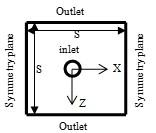Figure 3. Schematic of the computational domain for a single jet row; Top view of confinement plate

2.3 Boundary conditions

At the jet inlet, a constant velocity is prescribed. The target plate is modeled as a no-slip wall held at a constant temperature and all remaining channel walls were modeled as adiabatic no-slip walls. At the outlet, a pressure outlet boundary condition is used, i.e. prescribed pressure and zero velocity gradient.

2.4 Numerical

The numerical model is based on the solution of the stationary Reynolds-averaged Navier-Stokes equation with a finite volume method. This computational fluid dynamics (CFD) model is set up and run using the commercial code STAR-CCM 13.02.013 by CD-Adapco. The final solution was obtained by applying a second-order discretization upwind scheme for the pressure, momentum and energy terms, the SIMPLE algorithm is used for pressure-velocity coupling and a segregated flow solver was used for all the calculations. The turbulence is described by the two equation SST k-ω turbulence model as implemented in STAR-CCM. The flow in the near-wall region was simulated using a low-Reynolds number approach, leading to the requirement of a very fine mesh in the near wall region. The solution is considered to be converged for values of the scaled residual of the continuity, momentum, and energy equations smaller than 10-4 For further details with regard to the implementation of the numerical schemes, see Ref. .

2.5 Meshing

A polyhedral mesh is generated using the STAR-CCM+ auto-mesher. The grid is refined near the target wall in order to enable better resolution of the flow in this part as required by the low-Reynolds number approach and to appropriately describe the heat transfer in this region. Boundary layers with a y+ value less than one are built on the target plate of the model and y+ is defined as $y \sqrt{\tau_{w} / \rho} / v$ where τw is wall shear stress.

A mesh sensitivity analysis was carried out with a single jet row and for a high Reynolds number (Re= 23,000) in order to estimate the numerical accuracy of the CFD results. Three simulations with the same geometry but different grid sizes were carried out to check the grid independence of the simulation. The mesh sizes are summarized in Table 2.

Table 2. Grid parameters of the refinement study

 Grid Base Size (m) Cell Number Max y+ Average GCI % Course 0.000395 476561 0.493 --- Intermediate 0.000285 1049923 0.507 2.3 Fine 0.000205 2328819 0.510 1.65
The local discretization error distribution is calculated by applying the grid convergence index (GCI) method  to the Z-centerline Nusselt number distribution. The overall discretization error for the intermediate and coarse grid was small, see Table 2. The features of the secondary peak in the Nusselt number distribution are appropriately reproduced by the intermediate and the fine grid. In order to reduce the computational cost, the intermediate grid is chosen to perform the computations.
3. Results and Discussion

3.1 Evaluation of Computational Model

For numerical predictions of multi-jet impingement heat transfer, a quantification of the numerical accuracy is equally significant. Figure 4 shows the comparison of the total average Nu number for inline arrays at H/d = 5.4 and S/d=7.2. The average Nusselt numbers for different Reynolds numbers are also compared to the correlation presented by Martin . The trends and the slope of the CFD predictions are in agreement with the experiments, however, the level of heat transfer is overestimated (the average error is around 13%).

## 4.jpg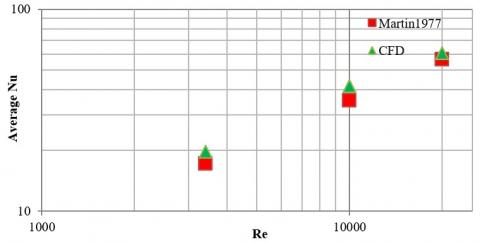Figure 4. Comparison of total average Nusselt numbers from experiments and CFD for inline arrays at H/d = 5.4, S/d=7.2 for different Reynolds numbers in the logarithmic scale

3.2 Number of jet rows

The velocity contours along the symmetry plane (X-Y plane in the middle of the jet array, Z=0) for a different number of jet rows are shown in Figure 5. There are two effects linked to the presence of additional rows: crossflow and jet to jet interaction. The presence of the crossflow will disturb the impinging jet flow pattern and thicken the wall boundary layers. The wall jets from adjacent jets interact with each other resulting in an upward flow from the wall. These fountains can cause recirculating flow zones, which then re-enter the neighboring jet cores and affect the impinging jet behavior, weaken the kinetic momentum and distort the impinging jet.

The crossflow degrades the heat transfer rates, due to the thickening of the wall boundary layers. The thermal exchange occurring between the recirculating flow and the impingement plate has also a negative effect on the target plate heat transfer.

## 5.png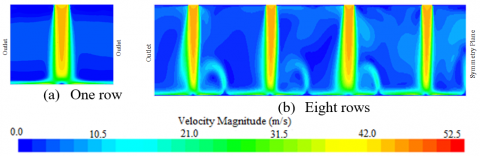Figure 5. Velocity magnitude contours along symmetry plane at H/d= 5.4, S/d=7.2, Re=10,000, for one row (a) and eight rows (b)

## 6.pngFigure 6. Pressure distribution on target surface at H/d= 5.4, S/d=7.2, Re=10,000 for one row (a) and eight rows (b)

Figure 6 shows the distributions of pressure on the target surface for the different numbers of jet rows. The pressure has the maximum value at the stagnation point. It reduces as the flow gets accelerated in the wall jet region. With an increasing number of rows, the wall jet interference leads to the asymmetry in the pressure distribution and a smaller value for pressure force. Thus the pressure force coefficient is also highest for a single row.

3.3 Jet Reynolds Number (Re)

A comparison of the total average Nusselt number between single and multiple rows is shown in Figure 7. The heat transfer depends on the Reynolds number. The correlation Nuave ~ Re 0.54 is independent of the number of rows. The level of heat transfer for multiple rows for these jet distances is lower than for the single row, e.g. for 8 rows by approximately 15%.

There is only a negligible dependency of the pressure force coefficient from the Reynolds number in the range examined, see Figure 8. The force coefficients for these jet distances are a little lower for multiple rows (on average 5% for 8 rows). Increasing the jet exit velocity from the nozzle leads to increasing pressure at the stagnation point on the target surface. Due to the definition of the force coefficient, the pressure force coefficients are relatively insensitive to the Reynolds number.

## 7.jpgFigure 7. Comparison of total average Nusselt numbers between single and multiple rows for inline arrays at H/d= 5.4, S/d=7.2 for different Reynolds numbers in the logarithmic scale

## 8.jpg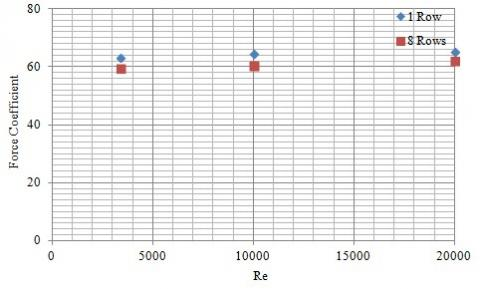Figure 8. Comparison of pressure force coefficient at H/d= 5.4, S/d=7.2 for different Reynolds numbers

3.4 Separation Distance (H/d)

The comparison of total average Nusselt number between single and multiple rows for different separation distance H/d at S/d=7.2and Re=10,000 is shown in Figure 9 and the corresponding pressure force coefficient is shown in Figure 10.

There are two regions: the region of small plate to nozzle distance, H/d < 2, where the flow in the impact zone is severely influenced by the confinement, and the region of bigger distances H/d >2, where the influence of the separation distance on the dimensionless numbers is the only minor.

For H/d >2, there is a slight decrease in Nusselt number with increasing distance. The correlation is weak for single as well as for multiple rows, with Nuave~(H/d)-0.016, and may be neglected for practical applications. The level of heat transfer from multiple rows is markedly lower (e.g. for 8 rows by approximately 20%) then from the single row.

For the minimum distance, different effects on heat transfer are predominant: when the separation distance is decreased, there is a notable enhancement of heat transfer for single rows. This is due to the secondary maximum peaks in the local Nusselt number distribution. For multiple rows with small separation distance, the jet interaction after impingement increases significantly and the resulting heat transfer rates can differ substantially from those of the single row. Here the negative effects of jet interaction of the intensified wall jets get predominant.

Figure 10 shows the comparison of pressure force coefficients for different separation distances (H/d). For H/d>2, there is a small decrease in the pressure force coefficient with increasing distance. There are similar values for single rows and multiple rows. The results may be correlated as Cf~(H/d)-0.093. Increasing the separation distance, the momentum exchange between the jet flow and the ambient increases, hence the flow in case of low H/d has more momentum compared to the higher H/d. Therefore, when H/d decreases, the pressure on the impingement plate increases. These results coincide with the findings  for a single impinging jet.

For smaller distances (i.e. H/d < 2) the pressure force coefficients increase with decreasing H/d due to the confinement effects and gets significant for very small distances (H/d < 1). Where the flow of the impingement jet is confined by smaller jet to target plate distance, the accumulations of cross-flows from sequential rows of jets can also result in local augmentation of pressure force in the vicinity of the stagnation point. The level of pressure force coefficients for multiple rows is thus also higher than for the single row. Therefore, the minimum distance is not appropriate for multiple jet rows and for products sensitive to pressure forces.

## 9.jpgFigure 9. Comparison of total average Nusselt numbers between single and multiple rows for inline arrays at S/d=7.2 and Re=10000 for different separation distance

## 10.jpgFigure 10. Comparison of pressure force coefficient from single and multiple rows at S/d= 7.2 and Re=10000 for different separation distance

3.5 Jet to Jet Spacing (S/d)

The comparison of the total average Nusselt number between single and multiple rows for the different jet to jet spacing is shown in Figure 11 and the comparison of the pressure coefficient is shown in Figure 13. The averaging of the Nusselt number is done over the total impingement surface area. Thus with increased spacing, the values of the corresponding surface area increase resulting in a decrease of the area-averaged dimensionless numbers. The pressure force coefficient is calculated based on the total area and is thus by definition also dependent on the spacing.

## 11.png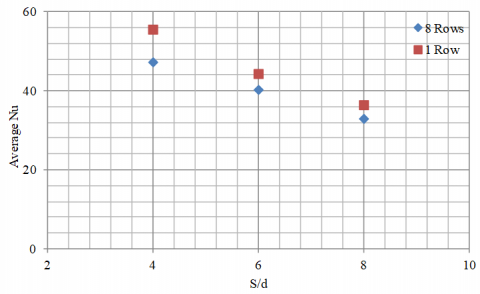Figure 11. Comparison of total average Nusselt between single and multiple rows at H/d= 5.4, Re=10000 for different jet-jet spacing

## 12.jpg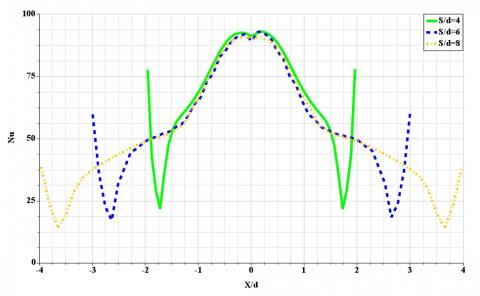Figure 12. Comparison of spanwise Nu distributions at H/d=5.4 and Re=10000 and different jet-to-jet distance (S/d)

## 13.pngFigure 13. Comparison of pressure force coefficient for single and multiple rows at H/d= 5.4, Re=10000for different jet-jet spacing

The trends and the slope of the computed Nusselt numbers are similar for both single and multiple rows and correlate as Nuave ~(S/d) -0.73. This decrease is not so pronounced as expected from the area averaging: Jet arrays with a smaller jet-to-jet spacing have a smaller surface area and thus a greater fraction of the impingement surface area is covered by the stagnation region with strong heat transfer, see Figure 12.

The level of heat transfer for multiple rows is lower than the heat transfer for a single row, e.g. for 8 rows by approximately 10%. With smaller jet-to-jet distances, the wall flows of the two jets are impinging upon each other and hence form a new stagnation region with higher heat transfer. This interaction is enhanced by additional jet rows.

Figure 13 shows the comparison of pressure force coefficients from single and multiple rows for the different jet to jet spacing (S/d) and the slope of the computed force coefficients is similar for both single and multiple rows. With Cf ~(S/d)2.2 the main influence is due to the consideration of the area in the definition of this dimensionless number Cf. There is a small influence of additional rows (average 10% for 8 rows), mainly due to the adjacent jet interference and crossflow effects from the multiple rows. As the distances increases, these effects get smaller.

3.6 Jet angle

A variation of the jet angle was performed for small distances H/d=1. The jet angle is varied between 45°, 60°, and 90° as measured from the target surface. The case of 90° corresponds to the orthogonal jet with maximum heat transfer and pressure force. With decreasing the jet angle the distance covered from jet exit to the plate increases. The orthogonal component of the jet flow decreases but on the other hand, the flow component parallel to the wall increases having the opposite effect. For multiple rows in addition to the cross-flow effects the adjacent jets significantly influence the flow in the impingement zone.

Figure 14 shows the comparison of the total averaged Nusselt number between single and multiple rows for different jet angles. The heat transfer decreases with increasing the inclination, i.e. with decreasing the jet angle. The trends and the slope of the computed values are similar for both single and multiple rows. Due to the small jet to surface distance, the values of the Nusselt number for multiple rows are smaller than for the single row. The Nusselt number correlates fairly with the sine of the jet angle. The influence of the jet angle is more distinct for small Reynolds numbers.

## 14.pngFigure 14. Comparison of total average Nu between single and multiple rows at H/d= 1, S/d=5 and Re=23000 for different jet angles

The Nusselt number distribution for different angles is shown in Figure 15. With increasing the inclination, the heat transfer peak in the impact zone gets more distinct and the distribution more asymmetric as the heat transfer decrease significantly on the side with reduced wall jet.

## 15.jpg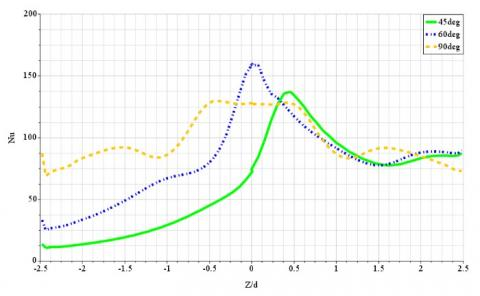Figure 15. Comparison of Nusselt number distributions in the exit flow direction for inline arrays at H/d=1, S/d=5 and Re= 23,000 for different jet angles

Figure 16 shows the comparison of pressure force coefficients from single and multiple rows for different jet angles. The pressure force coefficient for the single row correlates strongly with the orthogonal component of the jet, i.e. the sine of the jet angle.

## 16.jpg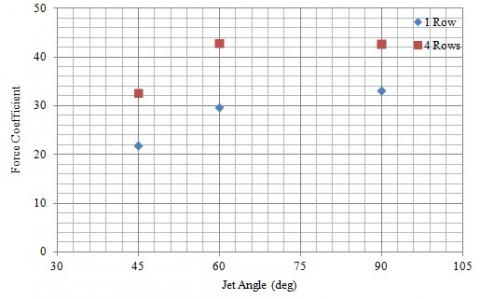Figure 16. Comparison of pressure force coefficient for single and multiple rows at H/d= 1, S/d=5 and Re=23000 for different jet angles

3.7 Surface motion

The movement of the surface is considered in the Z-direction, as shown in Figure 3, for a surface to plate distance of H/d=2. Due to the velocity of the plate (VPlate), the impinging jet is entrained in the direction of the moving plate, the wall-flow in the opposite direction is considerably restrained.

Figure 17 shows the distribution of the Nusselt number at the target surface at varying surface velocity. Increasing the surface velocity, the high heat transfer area, which originally occurs at the impinging zone, shifts downstream and the shape of the zone is also distorted in the downstream direction as the Nusselt number distribution gets asymmetric.

## 17.jpgFigure 17. Comparison of local Nunumber distributions in the direction of motion (Z) at H/d=2, S/d=10, and Re=23000

## 18.jpg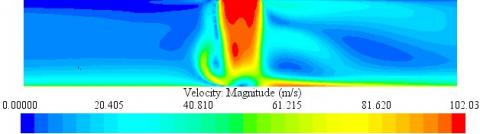Figure 18. Velocity magnitude contours in the symmetry plane at H/d= 2, S/d=10, Re=23000 and Vplate/Vj =1

At a high velocity ratio (Vplate/Vj=1.0), a negative local Nusselt number can be detected near the impingement region, see Figure 17, due to flow separation from the target surface, see Figure 18.

Figure 19 shows the comparison of total average heat transfer coefficients between single and multiple rows for different velocity ratio (Vplate/Vj). For surface velocities significantly smaller than the jet exit velocity (Vplate/Vj<0.1), the influence on the average Nusselt number is mostly negligible as the decrease of the heat transfer on one side is compensated by the increase on the other side. Further increasing the surface velocity leads to a decrease in the average Nusselt number Nuave~ (1+Vplate/Vj)0.85. This trend is more pronounced for multiple rows and lower Reynolds numbers. An increase in surface motion increases the interaction between neighboring jet flows and leads to significant reductions in local heat transfer rates. The flow in the impingement zone, associated with the maximum heat transfer, gets distorted, resulting in a reduction of the heat transfer. This disturbance is enhanced by the additional crossflow caused by additional rows.

## 19.jpgFigure 19. Comparison of total average Nusselt number between single and multiple rows at H/d= 2, S/d=10, and Re=23,000 for different velocity ratio

Figure 20 shows the comparison of pressure force coefficients from single and multiple rows for different velocity ratio (Vplate/Vj). The pressure force coefficient decreases with an increase in the surface velocity Cf~ (1+Vplate/Vj) -0.24. This is due to the entrainment of the impinging jet by the moving surface. The force coefficient for multiple rows is a little higher compared to a single row.

## 20.jpgFigure 20. Comparison of pressure force coefficient for single and multiple rows at H/d= 2, S/d=10 and Re=23,000 for different velocity ratio

4. Correlation Equations

The computed results for the parameter range of Table 1 show that with regard to heat transfer as well as pressure force the effect of additional jets and also small separation distance (H/d < 2) has to be considered. Some of the minor influencing factors as turbulence levels of jet or end effects were not taken into account. The resulting data for heat transfer on the surface for multiple impinging equidistant jets may be described by the following set of simple correlations valid for the parameter range of Table 1.

H/d ≥2 single row:

$\mathrm{Nu}_{\mathrm{ave}}=1.09 \mathrm{Re}^{0.54}(\mathrm{S} / \mathrm{d})^{-0.73}\left(1+\mathrm{V}_{\text {plate }} / \mathrm{V}_{\mathrm{jet}}\right)^{-0.85}$  (5)

The influence of multiple rows N on the reduction in average Nusselt number DNuave may be assessed by

$\mathrm{Nu}_{\mathrm{ave}, \mathrm{N}}=\mathrm{Nu}_{\mathrm{ave}}-\Delta \mathrm{Nu}_{\mathrm{ave}}$  (6)

$\Delta \mathrm{Nu}_{\text {ave }} / \mathrm{Nu}_{\text {ave }}=0.1233(\mathrm{N}-1)^{0.127}$ (7)

H/d <2 single row:

$\mathrm{Nu}_{\mathrm{ave}}=0.78 \mathrm{Re}^{0.54}(\mathrm{S} / \mathrm{d})^{-0.49}(\sin \theta)^{0.5}$  (8)

Multiple rows significantly change the coefficients, as for N=8:

$\mathrm{Nu}_{\text {ave }, 8}=0.85 \mathrm{Re}^{0.49}(\mathrm{S} / \mathrm{d})^{-0.46} \sin \theta$  (9)

The observed trends in the CFD simulations are shown by the exponents of the independent parameters in the correlations. The agreement between the CFD simulation and predicted results by correlations is found to be reasonable and all the data points deviate from the correlation by less than 6% for the average Nu number. This Nusselt number is in good agreement with other correlations from the literature in the common range of validity. The difference between the developed correlation and Martin correlation is approximately 10% on average.

5. Conclusions

Based on a CFD-model of impinging jets, the effects of row number, Reynolds number, separation distance, jet to jet spacing, jet angle and surface motion with regard to average heat transfer and pressure force were investigated.

A commercial CFD code with standard settings was used for the computations. The geometry was simplified by neglecting the end effects and using symmetry conditions. The k-omega SST model was used to describe the turbulence. The overall agreement with validation data and data from the literature was good, which showed that a reasonably simplified CFD model is generally suited for the prediction of complex impingement configurations. It is feasible to use this approach for the performance prediction of impinging jet arrays.

The computed results show that with regard to heat transfer as well as pressure force which is neglected in most investigations, the effect of additional jet rows has to be considered. The dependence on jet Reynolds number, jet to jet distance, jet to surface distance, jet angle and surface velocity for single and multiple rows were quantified. The results agree with the trends and the data from the literature, as far as available.

Correlation equations were derived using the CFD data. Nusselt number may be calculated for a wide range of significant parameters as used in many industrial applications with sufficient accuracy.

The investigation showed the occurrence of interesting flow phenomena: interacting jets for small distances (H/d < 2), recirculation in the impingement zone for high surface velocities (Vplate/V>0.2), the transition from impinging to wall jet with increasing jet inclination (jet angle < 80°). These flow regimes develop because the predominant mechanisms influencing the heat transfer and pressure force, change and transform. These effects get more significant as the number of the rows increases as also the interactions of multiple jets in an array increases. Thus with changing the surface velocity, array size and jet inclination, the geometric parameters for an optimum heat transfer are shifting. For design purpose these dependencies should be considered because increasing the surface velocity, adding additional rows or inconsiderately changing the jet geometry might lead to contrary results. In addition, if pressure force on the surface is of importance, the different dependencies of heat transfer and pressure force on the parameters must be taken into account.

In order to better assess the physical mechanisms and to give further insight into the complex flow phenomena for moving surfaces and jet inclination for a small distance of jet arrays to surface, further investigations based on DNS or LES computational models as well as additional experimental data in this parameter range would be beneficial.

Nomenclature
 Af open area ratio, total jet area to heat transfer area A surface area (m2) Cf force coefficient d jet diameter (mm) F force (N) H nozzle-to-target spacing (mm) kt thermal conductivity (W/mK) N Number of jet rows Nu Nusselt number P pressure (Pa) q convective heat flux (W/m2) Re Reynolds number S center-to-center distance (mm) S/d dimensionless spacing between jets Sx streamwise jet-to-jet distances Sy spanwise jet-to-jet distances T temperature ( oK) V = magnitude of jet exit velocity (m/s) X, Y, Z coordinates y+ dimensionless wall distance Greek symbols k turbulence kinetic energy (m2s-2) ω specific dissipation rate of turbulence kinetic energy (1/s) θ jet inclined angle with respect to the horizontal axes (deg) ρ density of the fluid (kg/m3) ν fluid kinematic viscosity (m2/s) Subscripts ave average j jet st stagnation point w wall Abbreviation CFD computational fluid dynamic GCI grid convergence index VR velocity ratio; surface to jet velocity SST shear stress transport
References

 Weigand, B., Spring, S. (2011). Multiple jet impingement− A review. Heat Transfer Research, 42(2): 101-142. https://doi.org/10.1615/HeatTransRes.v42.i2.30

 Zuckerman, N., Lior, N. (2006). Jet impingement heat transfer: Physics, correlations, and numerical modeling. Advances in Heat Transfer, 39: 565-631. https://doi.org/10.1016/S0065-2717(06)39006-5

 Bu, X., Peng, L., Lin, G., Bai, L., Wen, D. (2015). Experimental study of jet impingement heat transfer on a variable-curvature concave surface in a wing leading edge. International Journal of Heat and Mass Transfer, 90: 92-101. https://doi.org/10.1016/j.ijheatmasstransfer.2015.06.028

 Patil, V.S., Vedula, R.P. (2015). Heat transfer with single and two rows of axisymmetric jets impinging on a concave surface. 1st International ISHMT-ASTFE Heat and Mass Transfer Conference, Thiruvananthapuram, India.

 Wae-hayee, M., Yeranee, K., Piya, I., Rao, Y., Nuntadusit, C. (2019). Heat transfer correlation of impinging jet array from pipe nozzle under fully developed flow. Applied Thermal Engineering, 154: 37-45. https://doi.org/10.1016/j.applthermaleng.2019.03.044

 Li, Y., Li, B., Qi, F., Cheung, S.C. (2018). Flow and heat transfer of parallel multiple jets obliquely impinging on a flat surface. Applied Thermal Engineering, 133: 588-603. https://doi.org/10.1016/j.applthermaleng.2018.01.064

 Chandramohan, P., Murugesan, S.N., Arivazhagan, S. (2017). Heat transfer analysis of flat plate subjected to multi-jet air impingement using principal component analysis and computational technique. Journal of Applied Fluid Mechanics, 10(1): 293-306.

 Li, W., Li, X., Yang, L., Ren, J., Jiang, H., Ligrani, P. (2017). Effect of Reynolds number, hole patterns, and hole inclination on cooling performance of an impinging jet array—Part I: Convective heat transfer results and optimization. Journal of Turbomachinery, 139(4): 041002. https://doi.org/10.1115/GT2016-56205

 Li, W., Xu, M., Ren, J., Jiang, H. (2017). Experimental investigation of local and average heat transfer coefficients under an inline impinging jet array, including jets with low impingement distance and inclined angle. Journal of Heat Transfer, 139(1): 012201. https://doi.org/10.1115/1.4034165

 Ekkad, S., Huang, Y., Han, J.C. (2000). Impingement heat transfer measurements under an array of inclined jets. Journal of Thermophysics and Heat Transfer, 14(2): 286-288. https://doi.org/10.2514/2.6524

 Kamal, R.M., El sayed Mostafa, M., Aziz, S.S.A. (2006). An experimental study of an oblique multiple circular air jets impingement on a flat plate. Eight International Congress of Fluid Dynamics and Propulsion, Egypt.

 Attalla, M., Maghrabie, H.M., Specht, E. (2017). Effect of inclination angle of a pair of air jets on heat transfer into the flat surface. Experimental Thermal and Fluid Science, 85: 85-94. https://doi.org/10.1016/j.expthermflusci.2017.02.023

 Chattopadhyay, H. (2006). Effect of surface motion on transport processes due to circular impinging jets—A numerical study. Drying Technology, 24(11): 1347-1351. https://doi.org/10.1080/07373930600951117

 Badra, J., Masri, A.R., Behnia, M. (2013). Enhanced transient heat transfer from arrays of jets impinging on a moving plate. Heat Transfer Engineering, 34(4): 361-371. https://doi.org/10.1080/01457632.2013.717046

 Kadiyala, P.K., Chattopadhyay, H. (2017). Numerical simulation of transport phenomena due to array of round jets impinging on hot moving surface. Drying Technology, 35(14): 1742-1754. https://doi.org/10.1080/07373937.2016.1275672

 Wang, X.K., Niu, G.P., Yuan, S.Q., Zheng, J.X., Tan, S.K. (2015). Experimental investigation on the mean flow field and impact force of a semi-confined round impinging jet. Fluid Dynamics Research, 47(2): 025501. https://doi.org/10.1088/0169-5983/47/2/025501

 Penumadu, P.S., Rao, A.G. (2017). Numerical investigations of heat transfer and pressure drop characteristics in multiple jet impingement system. Applied Thermal Engineering, 110: 1511-1524. https://doi.org/10.1016/j.applthermaleng.2016.09.057

 El-Gabry, L.A., Kaminski, D.A. (2005). Numerical investigation of jet impingement with cross flow—Comparison of yang-shih and standard k–ϵ turbulence models. Numerical Heat Transfer, Part A, 47(5): 441-469. https://doi.org/10.1080/10407780590891254

 Hebert, R., Ekkad, S.V., Gao, L., Bunker, R.S. (2005). Impingement heat transfer, Part II: Effect of streamwise pressure gradient. Journal of Thermophysics and Heat Transfer, 19(1): 66-71. https://doi.org/10.2514/1.8588

 Suzuki, T., Tsujimoto, K., Shakouchi, T., Ando, T. (2018). DNS of flow and heat transfer characteristics of multiple impinging jets. 21st Australian Fluid Mechanics Conference, Adelaide, Australia.

 Draksler, M., Končar, B., Cizelj, L., Ničeno, B. (2017). Large Eddy Simulation of multiple impinging jets in hexagonal configuration–Flow dynamics and heat transfer characteristics. International Journal of Heat and Mass Transfer, 109: 16-27. https://doi.org/10.1016/j.ijheatmasstransfer.2017.01.080

 Martin, H. (1977). Heat and mass transfer between impinging gas jets and solid surfaces. In Advances in Heat Transfer, 13: 1-60. https://doi.org/10.1016/S0065-2717(08)70221-1

 Siemens PLM. STAR-CCM+. https://www.plm.automation.siemens.com/, accessed on Jan. 15, 2018.

 Roache, P.J. (1994). A method for uniform reporting of grid refinement studies. Journal of Fluids Engineering, 116: 405-413. https://doi.org/10.1115/1.2910291